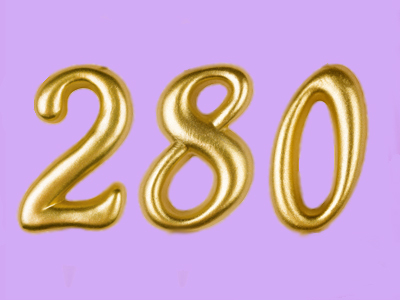280 lies exactly halfway between 200 and 360.

# Compare and Order Numbers 3

This Math quiz is called 'Compare and Order Numbers 3' and it has been written by teachers to help you if you are studying the subject at elementary school. Playing educational quizzes is an enjoyable way to learn if you are in the 3rd, 4th or 5th grade - aged 8 to 11.

It costs only \$12.50 per month to play this quiz and over 3,500 others that help you with your school work. You can subscribe on the page at Join Us

Ordering numbers is an important skill for the following reasons: you need to be able to arrange numbers in ascending and descending order, and you need to be able to understand what is meant by the magnitude (size) of a number.

Of course, when learning math you'll realize it's mostly about numbers. However, there are also mathematical terms that you won't come across elsewhere and these need to be understood in order to complete some calculations. As you move up the grades at school, be prepared to learn new words relating to math all the time.

1.
What is wrong with this calculation: 0 + 4 - 4 = 8?
0 + 4 - 4 = 0
0 + 4 - 4 = 4
0 + 4 - 4 = -4
0 + 4 - 4 = -8
-4 + 4 = 0. Whenever you have two identical numbers that differ only in sign - they cancel each other out to give zero
2.
How many times bigger than 2 is 16?
4
6
32
8
16 ÷ 2 = 8, so 16 is 8 times bigger than 2 because there are 8 lots of 2 in 16: 2 + 2 + 2 + 2 + 2 + 2 + 2 + 2 = 16
3.
What number lies exactly halfway between 200 and 360?
260
300
280
270
Here's how you solve problems like this: add both numbers together THEN divide by two: (200 + 360) = 560. So, 560 ÷ 2 = 280
4.
Starting from zero, if you keep on moving right along the number line, what happens to the numbers?
They become smaller and smaller negative numbers
They become larger and larger positive numbers
They finish quickly
They stop at zero if you go far enough
Let's hope that you didn't get this wrong! Remember that going RIGHT means positive numbers increasing. If you move LEFT starting from zero along the number line, the numbers become smaller and smaller negative numbers
5.
Which number lies exactly between -50 and 50?
0
100
55
45
-50 + 50 = 0. Whenever you have two identical numbers that differ only in sign, they are at the same distance from zero on either side of the number line: zero then lies exactly in the middle
6.
How would you arrange the following numbers in descending order: 2, 7, -4, 6, 0?
2, -4, 0, 6, 7
0, 2, -4, 6, 7
7, 6, 2, 0, -4
-4, 0, 2, 6, 7
'Descending order' means that the numbers decrease in size as you read from left to right
7.
Which of the following numbers is the largest: -6, 6, 0, -12?
-6
-12
0
6
6 is the only number greater than zero, so it is the largest
8.
Which of the following is the biggest number: -2, -22, 0, -222?
0
-2
-222
-22
All the other numbers are negative, so zero is the biggest number. ZERO IS A NUMBER
9.
How would you arrange the following numbers in ascending order: 3, 7, 1, 0, -3?
-3, 0, 1, 3, 7
0, -3, 1, 3, 7
1, 0, 3, -3, 7
7, 3, 1, 0, -3
'Ascending order' means that the numbers increase in size as you read from left to right
10.
How many times smaller than 27 is 3?
6
9
15
5
27 ÷ 3 = 9, so 27 is 9 times bigger than 3 because there are 9 lots of 3 in 27: 3 + 3 + 3 + 3 + 3 + 3 + 3 + 3 + 3 = 27
Author:  Frank Evans